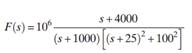### Create an Account

Home / Questions / The Dominant Pole Approximation When a transform F(s) has widely separated poles, then those..

# The Dominant Pole Approximation When a transform F(s) has widely separated poles, then those..

The Dominant Pole Approximation When a transform F(s) has widely separated poles, then those closest to the j-axis tend to dominate the response because they have less damping. An approximation to the waveform can be obtained by ignoring the contributions of all except the dominant poles. We can ignore the other poles simply by discarding their terms in the partial fraction expansion of F(s). The purpose of this example is to examine a dominant pole approximation of the transform(a) Construct a partial-fraction expansion of F(s) and find f (t).

(b) Construct a pole-zero diagram of F(s) and identify the dominant poles.

(c) Construct a dominant pole approximation g (t) by discarding the other poles in the partial fraction expansion in part (a).

(d) Plot f(t) and g (t) and comment on the accuracy of the approximation.

Jul 27 2020 View more View LessSubscribe To Get Solution